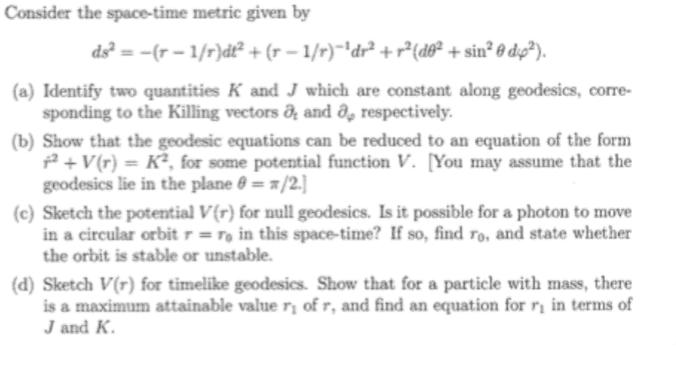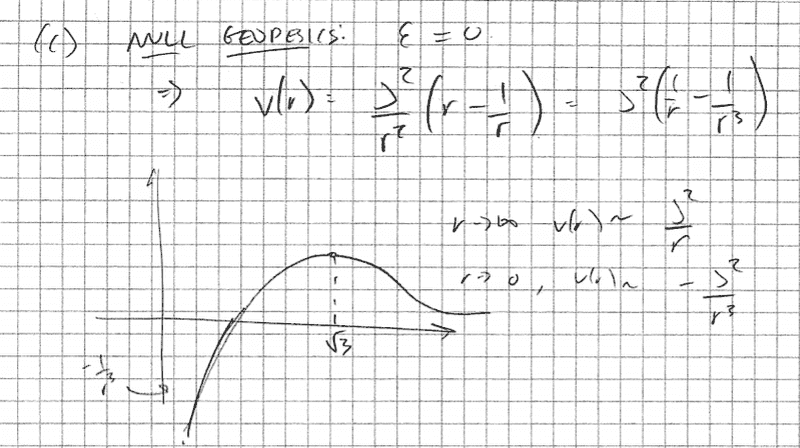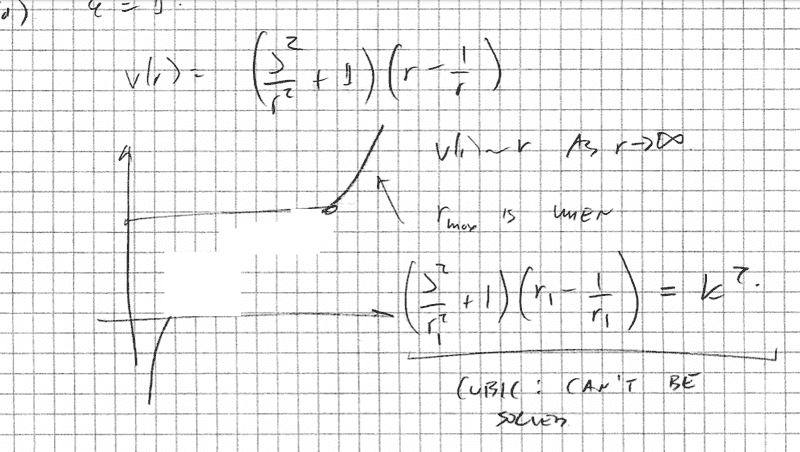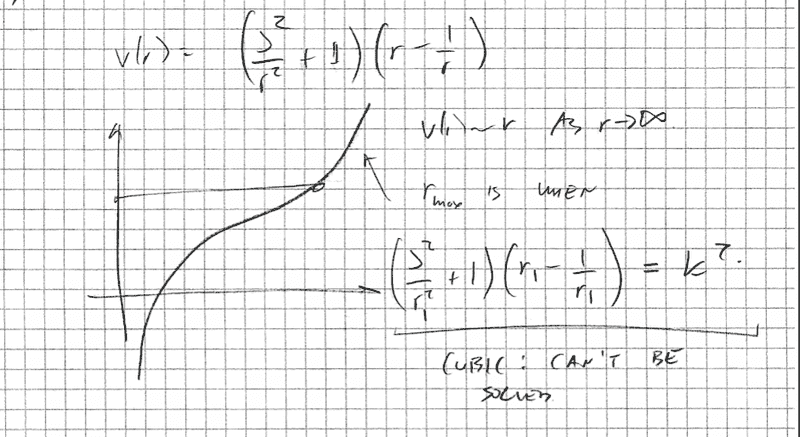# GR- Energy conservation, effective potential graph sketch

• binbagsss
In summary, the conversation discussed the graph sketching for parts c) and d) of a given equation. The solution involved using the critical point and checking for stability to determine the shape of the curve. However, for part d), the critical point was unsolvable, leading to uncertainty about the shape of the curve in that section. The conversation also mentioned a possible error in the solution regarding the value of epsilon.

## Homework StatementI would like to ask about parts c) and d) , the graph sketching bits.

2. Homework Equations

##V(r) = ( \frac{J^2}{r^2}+\epsilon)(r-\frac{1}{r}) ##

where the value of ## \epsilon ## is set according to whether time-like or null etc.

## The Attempt at a Solution

Q1)for part c)

##V(r) = ( \frac{J^2}{r^2})(r-\frac{1}{r}) ##

I am able to deduce the shape of the curve from the fact that the potential ##V'(r)=0 ## is a quadratic and solveable, and so i can check whether this is a stable or unstable point. So to sketch I:
a) ## \lim r \to \infty ## and ## \lim r\to 0 ##
b) to deduce the cts shape consider the critical point and check whether it is stable or unstable.
and that gives me the following which is fine.

(giving me the following:)

however for part d) instead we have ##V(r) = ( \frac{J^2}{r^2}+1)(r-\frac{1}{r}) ## and now ##\lim r \to \infty = r ## but ##\lim r \to 0 = -1/r^3 ##. from this I can draw the start and end , but am unsure how to connect in the middle- curving upward or downward. compared to the above, null example, where the critical point is solvable and i can check stability to deduce the shape, here the critical point is unsolvable so I am unsure how to do this.

I have the following so far:I AM UNSURE WHY, how to deduce the shape on the 'jointy' bit.

(I believe there may be an error in the solution since for a time-like geodesic instead of ##\epsilon=1 ## it should be ##\epsilon=-1## however I am interested in the principal of the graph sketching, so, assuming the graph sketch is consistent with the value of epsilon chosen for the solution, I'd like to ignore this please and focus on the graph sketching. Thanks).

Last edited:
bump
many thanks